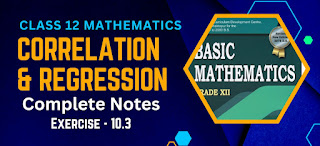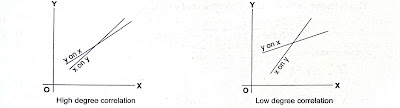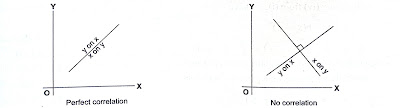# Correlation and Regression Analysis Class 12 Mathematics Solutions | Exercise - 10.3Correlation and Regression Analysis Class 12 Mathematics Solutions according to the latest syllabus of 2080. Many students were searching for the notes, so we are here to provide you the complete solutions of correlation and regression chapter. You can also download the PDF if you want to save the notes for future use.

## Chapter - 10  Correlation and Regression

In correlation and regression chapter we have to study about many different tooics. Since this chapter is divided in 3 exercises, we will study different topics in different exercises. In our previous exercise we have discussed about regression coefficient, nature of correlation, rank correlation and many more.

In this exercise, we will be discussing about regression, difference between regression and correlation, regression equation, lines of regression and properties of regression coefficient. So let's get into the context.

### Regression

The simple meaning of regression is returning back to the original position. The theory of regression was first developed by Sir F. Galton. He studied the heights of a group of father and their sons. He found that the taller fathers have taller sons and dwarf fathers have dwarf sons.

He also found that the average height of the sons of the taller father is less than the average height of the taller fathers and the average height of the dwarf sons is greater than the average height of their fathers. But now-a-days, regression analysis is used as a tool to present the strength of the relationship between the two variables.

Thus it is considered as a device which is used to predict the value of one variable when the value of other variable is known. The variable whose value is known is the independent variable and the variable whose value is to be determined is known as the dependent variable.

The analysis which is used to describe the average relationship between the two variables is known as a simple linear regresion analysis.

Exercise - 10.3

This PDF contains only contains the complete notes of 3rd exercise of correlation and regression chapter. If you want the notes of 1st and 2nd exercises then you can get them above.

Note: Scroll the PDF to view all solutions

### Difference between correlation and regression

The following are the differences between the correlation and regression.

1. Correlation means the relationship between the two variables so that the change in the value of one variable results change in the value of the other variable. But the regression means the returning back to the average value.
2. There is no need of cause and effect relationship between the two variables in case of correlation but there must be cause and effect relationship between two variables in case of regression.
3. Correlation analysis presents the extent to which the two variables are correlated and also the direction of their movements. But regression analysis aims to study the nature of the relationship between the two variables so that we may able to find the value of one variable when the value of other variable is known.
4. Correlation coefficient is independent of change of origin and scale but regression coefficients are independent of change of origin but not of scale.
5. The correlation coefficient between the two variables can not exceed 1 but one regression coefficient can exceed 1 and other regression coefficient less than 1 making their product less than or equal to 1.

### Lines of regression

Whenever there shows a relationship between the two variables, the dots of the scatter diagram will concentrate around a certain curve. If the curve is a straight line, then it is known as the line of regression.

A line of regression gives the best estimate (in the sense of least square method) of one variable for a given value of the other variable. So, there are in general two lines of regression. One is the line of regression of y on x giving the best estimated value of y whenever the value of x is known. Other is the line of regression of x on y giving the best estimated value of x.

Whenever the value of y is known. The two lines of regression intersect at the point (x, y), x and y being the averages of x and y. If there is a wide gap between the two lines of regression, the correlation between the two variables is less. If the two lines of regression are near enough, then the correlation between the two variables is high.Whenever the two lines of regression coincides there is a perfect correlation between the two variables. On the other hand, if the two lines of regression intersect at right angles, there is no correlation between the two variables### Regression Equations

Regression lines expressed in terms of algebraic relations are known as the regression equations. Since there are two regression lines, so there are two regression equations.

The regression equation of y on x expresses the variation of y for a change in the value of x. The regression equation of x on y expresses the variabtion of x for a change in the value of y.

### Properties of regression coefficients

Regression coefficients follow the following properties:

1. The regression coefficients are independent of change of origin but not of scale.
2. The correlation coefficient between the two variables is the geometric mean between the two regression coefficients.
3. The product of the two regression coefficient is less than or equal to 1. The two regression coefficients have the same sign.
4. The sign of the correlation coefficients depends upon the sign of the regression coefficients. That is, if both regression coefficients are positive, the correlation coefficient is positive and if both regression coefficients are negative, then the correlation coefficient is negative.
5. The arithmetic average of the two regression coefficients is greater than or equal to the correlation coefficient.
What is regression?

The simple meaning of regression is returning back to the original position.

What is the difference between correlation and regression?

Correlation means the relationship between the two variables so that the change in the value of one variable results change in the value of the other variable. But the regression means the returning back to the average value.

What are the lines of regression?

Whenever there shows a relationship between the two variables, the dots of the scatter diagram will concentrate around a certain curve. If the curve is a straight line then it is known as the lines of regression.

Why are there in general two lines of regression?

A line of regression gives the best estimate of one variable for a given value of the other variable. So there are in general, two lines of regression. One is the line of regression of y on x giving the best estimated value of y whenever the value of x is known. Other is the line of regression of x on y giving the best estimated value of x whenever the value of y is known.

No Comment